Courses

# Test: Polygons

## 15 Questions MCQ Test UPSC Prelims Paper 2 CSAT - Quant, Verbal & Decision Making | Test: Polygons

Description
This mock test of Test: Polygons for UPSC helps you for every UPSC entrance exam. This contains 15 Multiple Choice Questions for UPSC Test: Polygons (mcq) to study with solutions a complete question bank. The solved questions answers in this Test: Polygons quiz give you a good mix of easy questions and tough questions. UPSC students definitely take this Test: Polygons exercise for a better result in the exam. You can find other Test: Polygons extra questions, long questions & short questions for UPSC on EduRev as well by searching above.
QUESTION: 1

Solution:
QUESTION: 2

### What is the measure of each interior angle of a regular hexagon (in degrees)?

Solution:

To find the sum of the interior angles of a hexagon, divide it up into triangles. There are four triangles.

Because the sum of the angles of each triangle is 180 degrees.

4 x 180° = 720°

So, the sum of the interior angles of a hexagon is 720 degrees.

All sides are the same length (congruent) and all interior angles are the same size (congruent).

To find the measure of the interior angles, we know that the sum of all the angles is 720 degrees. And there are six angles.

720° ÷ 6 = 120°

So, the measure of the interior angle of a regular hexagon is 120 degrees.

QUESTION: 3

### The figure below is a regular polygon. Find the measure of angle x (in degrees)?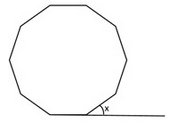Solution:
QUESTION: 4

If ABCDEF is a regular hexagon and AB = 1cm, then what is the area of this regular hexagon ABCDEF?

Solution:

formula for finding the area of a hexagon is Area = (3√3 s2) / 2 where s is the length of a side of the regular hexagon

where s = 1

Hence area of hexagon will be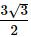QUESTION: 5

The area of polygon ABCD is equal to 8√3 cm2. If triangle ABD and BCD are equilateral triangles, then find the perimeter of the polygon ABCD?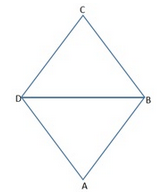Solution:
QUESTION: 6

ABCDE is a regular pentagon. Sides AE and ED of the pentagon are extended as shown to form triangle EFG, which is right-angled at F. Vertices A and C are joined to form the triangle ABC. What is the value of sum (x° + y°)?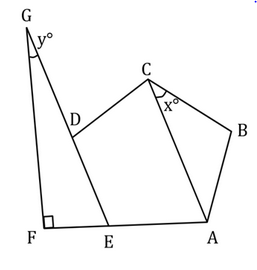Solution:

Step 1: Understand the question and draw inferences

To find –x°+ y°

Given – ABCDE is a regular pentagon.

This means, all sides of the pentagon are equal.

Therefore, AB = BC

In triangle ABC, two sides are equal.

Therefore, triangle ABC is an isosceles triangle.

Since angles opposite to equal sides are also equal,

Angle BAC = Angle ACB = x°

Let Angle ABC = i°

Therefore, by Angle Sum Property,

2x° + i° = 180°   .  .  . (1)

Now, considering the right triangle EFG,

Let angle FEG = e°

Therefore, by Angle Sum Property,

e° + y° + 90° = 180°

e° + y° = 90°    .  .  . (2)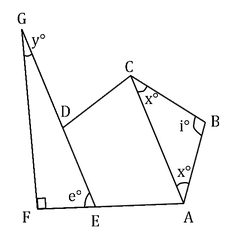Step 2: Finding the required values

We know that for interior angle of an n-sided regular polygon =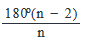So, for n = 5, value of each interior angle =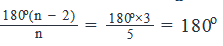Therefore, i° = 108°    . . . (3)

Also, we know that Exterior Angle of an n-sided regular polygon = 360on

So, for n = 5, the value of each exterior angle = 360o/5 =  72°

Notice that Angle FEG is an exterior angle of the regular pentagon.

Therefore, e° = 72°   .  .  . (4)

Step 3: Calculating the final answer

From (1) and (3), we get:

2x° + 108° = 180°

Solving this equation, we get x° = 36°

From (2) and (4), we get:

72° + y° = 90°

y° = 18°

Thus, x° + y° = 36° + 18° = 54°

Correct Choice -C

QUESTION: 7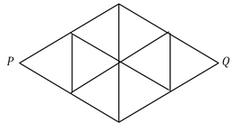The above shape is made of eight identical equilateral triangles, each having a perimeter of 3. What is the straight-line distance between points P and Q?

Solution:

Step 1: Question statement and Inferences

To find the straight line distance between points P and Q, calculate the height of one of the equilateral triangles.

Step 2: Finding required values

Think of the shape as two large, equilateral triangles. If each small equilateral triangle has a perimeter of 3, then it has a side of 1, and each large equilateral triangle has a side of 2.

Create a right triangle inside a large equilateral triangle, and find the third side, shown as h in the drawing.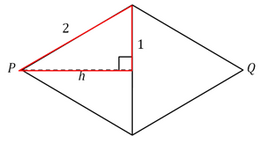Step 3: Calculating the final answer

The right triangle has sides 1, 2, and h, where 2 is the hypotenuse. With the Pythagorean Theorem, calculate the length of h: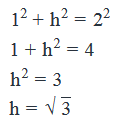You have found half the distance. Double this for the full straight-line distance of 2√3

QUESTION: 8

Corners of an equilateral triangle were cut off such that a regular hexagon is formed. If each side of the original triangle measures 9 cm, find the area of the hexagon in square cm.

Solution:

Step 1: Question statement and Inferences

Since we are not given the figure, let us draw one.

Let the equilateral triangle be ABC.

∠BAC = ∠ABC = ∠BCA = 600

The corners marked in blue are cut from this triangle, thereby resulting in a regular hexagon PQRSTU.

Let each side of this hexagon be x cm

Now, we know that

Exterior angle of a n-sided regular polygon  = 360o/n

Therefore,

Exterior angle of a regular hexagon

360o/6 = 60o

This means, Angle APQ = 60o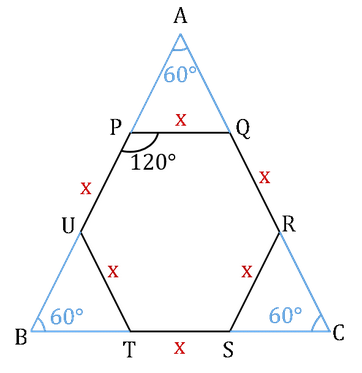Consider Triangle APQ:

Every angle in this triangle is 60o

Therefore, BU = UP = PA = x cm

So, BA = BU + UP + PA = 3x cm

But, we are given that

Each side of triangle ABC measures 9 cm

That is, BA = 9 cm

3x = 9 cm

x = 3 cm

Thus, we have found out that each side of the regular hexagon measures 3 cm.

We need to find the area of this regular hexagon.

Step2: Finding required values

Area of hexagon = (Area of equilateral triangle ABC) -3(Area of equilateral Triangle with side 3 cm)

Area of am equilateral triangle =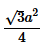; where a is the side of the triangle

so, the area of triangle ABC =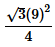And, the area of each smaller triangle =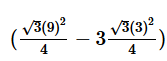Area of the hexagon =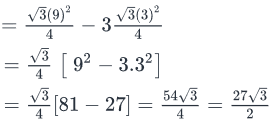Step 3: Calculating the final answer

So, the area of the hexagon is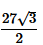square cm.

QUESTION: 9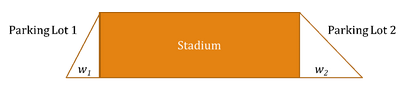Dodger’s stadium, which is rectangular in shape, currently has one parking lot that has a maximum capacity of 2000 cars.  In order to accommodate the increased demand due to District Level games, the management plans to increase the capacity of the parking lot by adding one more parking lot – parking lot 2.  Assuming the shape of the structure as shown in the figure, what is the ratio of the width of parking lot 2 (w2) to the width of parking lot 1(w1).  Also assume that the height of both the triangular parking lots is same as the width of the rectangle and that the allowed spacing between the cars is same in both parking lots.

(1) After the addition of parking lot 2, the total parking capacity of the stadium is 6000 cars.

(2) The area of parking lot 1 is 10000 m2

Solution:

Steps 1 and 2 – Understand the question statement and draw inferences

To find – w2 : w1

Given –

(1) Capacity of Parking Lot 1 = 2000 cars

(2) Per the figure, height of both parking lots is the same.  The difference in capacity of the parking lots comes from the difference in the width of the parking lots.

Step 3 – Analyse Statement 1

Per statement 1, the total increased capacity of the parking lots is 6000 cars.

• Capacity of Parking Lot 1 = 2000
• Capacity of Parking Lot 2 = 6000 – 2000 = 4000 cars

Area Lot 2 = 2 * Area Lot 1 … (since capacity of parking lot 1 = 2000 cars and that of lot 2 = 4000 cars)

Since the height of both parking lots is the same, the ratio of area = the ratio of width

Thus, we have the value of w2 : w1

Therefore, information provided in Statement (1) is sufficient to arrive at a unique answer.

Step 4 – Analyse Statement 2

Statement 2 provides area of Parking Lot 1 – 10000 m2.

So we know that the given area can accommodate 2000 cars.  But we do not know anything about the area of the parking lot 2.  Thus, we do cannot find the relationship between the widths of the two parking lots.

Therefore, statement (2) does not provide sufficient information to arrive at a unique answer.

Step 5 – Analyze both Statements together

This step is not required since statement 1 helps us arrive at a unique answer.

QUESTION: 10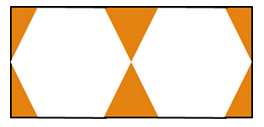Two  identical regular hexagons are inscribed in a rectangle whose area is 256√3 square inches.  What is the perimeter of each hexagon in inches?

Solution: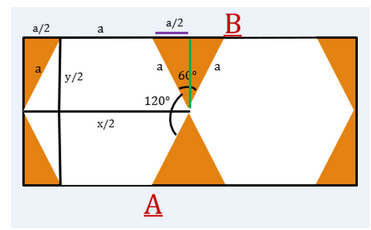To find – Perimeter of hexagon

Given –

• The two hexagons are identical – the length of the sides are equal.
• Area of rectangle = 256√3 in2

Approach –

• Let ‘a’ be the side of the hexagon and let ‘x’ be the length of the rectangle.
• To find the perimeter of the hexagon, we need to express the sides of rectangle (x) in terms of the side of the hexagon (a).
• Then given the area we can find the measure of ‘a’
• Hence, we can find the perimeter.

Solution

• Each side of the hexagon is ‘a’ since it is a regular hexagon.
• Consider the middle shaded triangle.
• It is an equilateral triangle since each angle is 60° as explained below.
• Interior angle of the hexagon = 120°.
• The angle made by AB = 180°.
• Thus, angle of the triangle = 180 – 120 = 60°
• The purple line is a/2.
• x/2 = a/2+a+a/2 = 2a
• x = 4a

Likewise, applying Pythagoras theorem on the shaded side triangle we get the value of y in terms of a

• a2-a2/4 = y2/4
• y = a√3

We can now substitute the values of x and y in terms of a in the equation for area of the rectangle.

• xy = 256√3
• 4a2√3 = 256√3
• a = 8
• Perimeter of hexagon = 8 * 6 = 48 inches

QUESTION: 11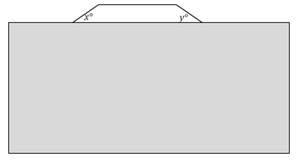A regular polygon is partially obscured by a box. What is the sum of the angles of the polygon?

(1) x°= y°

(2) x°+ y°= 72°

Solution:

Steps 1 & 2: Understand Question and Draw Inferences

To find the sum of the angles of the polygon, you need to know the number of angles.

Knowing that the polygon is regular, the two visible angles (and all the other hidden angles) are equal. You also know that the visible trapezoid has an angle total of 360°

Step 3: Analyze Statement 1

x°= y°

Knowing x equals y doesn’t tell you anything about the angles of the regular polygon.

Statement (1) is not sufficient.

Step 4: Analyze Statement 2

x°+ y°= 72°

The part of the regular polygon that is visible forms a quadrilateral.

This quadrilateral has 4 angles: x°, y° and two interior angles of the regular polygon.

Let each interior angle of the regular polygon = i°

Since the sum of all angles of a quadrilateral is 360°, we can write:

x° + y° + 2i° = 360°

Inserting the information given in Statement 2 in this equation, we get:

72° + 2i° = 360°

Or, 2i° = 288°

i° = 144°

Thus, we now know that every interior angle of the regular polygon measures 144°

Let the regular polygon have n sides.

Then 144°*n = (n -2)*180°

⇒ n = 10.

Therefore, sum of angles of the regular polygon = (n-2)180° = 8*180° = 1,440°.

Statement (2) is sufficient.

Step 5: Analyze Both Statements Together (if needed)

You get a unique answer in step 4, so this step is not required

QUESTION: 12

If the sum of interior angles of a polygon is 900 degrees, what is the number of diagonals it has?

Solution:

The sum of interior angles for an ‘n’ sided polygon is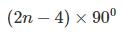If this value is 900, then the polygon is a heptagon.
The number of diagonals for an ‘n’ sided polygon =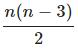= 14 for a heptagon.

QUESTION: 13

In a regular pentagon PQRST, what is the ratio of the area of triangle PRS to the area of the pentagon PQRST?

Solution:

Each angle of a regular pentagon is 108 degrees. In triangle PTS, angle T = 108 degrees, and PT = TS. So, angle TPS = angle TSP = 36 degrees.
Similarly, angle QPR = 36 degrees. So, angle SPR = 108 – (36 + 36) = 36 degrees
Also, PS = PR
Area of triangle PSR = ½ * PS * PR * sin 36
Area of the pentagon = Sum of areas of the three triangles = Area of triangle PTS + Area of triangle PSR + Area of triangle PQR
= ½ * PT * PS * sin (angle TPS) + ½ * PS * PR * sin 36 + ½ * PQ * PR * sin (angle QPR)
= ½ * PT * PS * sin 36 + ½ * PS * PR * sin 36 + ½ * PQ * PR * sin 36
Required ratio = ½ * PS * PR * sin 36 / (½ * PT * PS * sin 36 + ½ * PS * PR * sin 36 + ½ * PQ * PR * sin 36)
= PR / (PT + PR + PQ)
PT = PQ = SR
SR = 2 * PR cos (angle PSR) = 2 * PR * cos 72
Ratio = PR / (2 * PR cos 72 + PR + 2 * PR cos 72) = 1 / (4 cos 72 + 1)

QUESTION: 14

A regular polygon P has 135 diagonals. Find the exterior angle of the polygon P.

Solution:

Number of diagonals in a regular polygon =nC2–n
=>nC2–n

= 135
=> n = 18
Exterior angle = 360/n
=360/18
=200

QUESTION: 15

A cylindrical pencil consists of a layer of wood surrounding a solid cylinder of graphite. The radius of the pencil is 6 mm, the radius of the graphite cylinder is 2 mm and the length of the pencil is 10 cm. Find the cost of the material used in a pencil, if the cost of wood is Rs. 0.70/cm3 and that of graphite is Rs. 2.80/cm3.

Solution:

Volume of the whole pencil = 3.6π cm3
Volume of graphite cylinder =0.4π cm3
Volume of wood part =3.6π−0.4π=3.2π cm3

Cost of the pencil =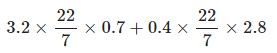= Rs. 10.56